# Applications of approximate algebraic geometry in industrial computer aided geometry

## Objective

The goal of the project is to evaluate to what extent approximate algebraic geometry can be used in industrial CAGD (computer aided geometric design). The project involves several mathematical domains that can be seen as complementary algebraic geometry, approximation theory and numerical geometry. The project will consist of a case study concerning the detection and treatment of various forms for intersections between geometrical objects like curves and surfaces. From an industrial point of view, the detection of intersections costs traditionally a lot of computation time and the treatment of self-intersections is almost non-existing. This means that one can gain considerable performance by introducing methods that decrease some of the expenses and leave more freedom to designers. We consider intersection techniques based on approximation by implicit curves and surfaces. They should be tested out in order to treat intersections.
The goal of the project is to evaluate to what extent approximate algebraic geometry can be used in industrial CAGD (computer aided geometric design). The project involves several mathematical domains that can be seen as complementary algebraic geometry, approximation theory and numerical geometry. The project will consist of a case study concerning the detection and treatment of various forms for intersections between geometrical objects like curves and surfaces. From an industrial point of view, the detection of intersections costs traditionally a lot of computation time and the treatment of self-intersections is almost non-existing. This means that one can gain considerable performance by introducing methods that decrease some of the expenses and leave more freedom to designers. We consider intersection techniques based on approximation by implicit curves and surfaces. They should be tested out in order to treat intersections.

OBJECTIVES
The goal of the project is to evaluate to what extent approximate algebraic geometry can be used in industrial CAGD. The project involves several mathematical domains that can be seen as complementary algebraic geometry, approximation theory and numerical geometry (CAGD). The project will consist of a case study concerning the detection and treatment of intersections as well as self-intersections between geometrical objects like curves and surfaces. From an industrial point of view, the detection of intersections costs traditionally a lot of computation time and the treatment of self-intersections is almost non-existing. This means that one can gain a considerable performance by introducing methods that decrease some of the expenses and leave more freedom to designers. If the study is promising for a further industrial development of similar methods, we consider this Assessment-project as a pre-project for a possible full RTD-project.

DESCRIPTION OF WORK
Detection of intersections between different geometric objects and of self-intersections (i.e. the curve or the surface cuts itself) is a large and important issue in the area of CAGD. Since traditional methods for finding intersections between two parameterised objects are based on iterative approaches, these are usually not very fast. We have thus chosen to consider intersection techniques based on an approximation by implicit curves and surfaces. That is, given a parameterised curve g(t) (with t as a parameter in a given interval), one constructs a polynomial F such that F(g(t))~0. We propose to develop a prototype toolbox with methods based on approximate implicitisation.
The methods are to be tested out on industrial examples, and they should treat the following cases:
1. Ordinary intersections. The objective is to decide whether two geometrical objects intersect within a given tolerance or not. The problem is as follows: given two parametric surfaces, we will detect possible intersections y approximating one of them by an implicit surface, F(x,y,z)=0.
2. Near-intersections. Methods to detect cases when two objects intersect clearly in one point, but there are ambiguities in the surrounding area (tangency or higher order contact).
3. Self-intersections and singularities. This should be considered as another kind of separation methods, but now we only consider one curve or surface. With classical techniques, we can detect e.g. singularities in implicit objects without iteration. The main points in the work plan are mathematical classification of implicit representations, development and implementation of numerical algorithms, validation of the numerical algorithms and validation and integration of the numerical industrial results.

### Call for proposal

Data not available

### Funding Scheme

ACM - Preparatory, accompanying and support measures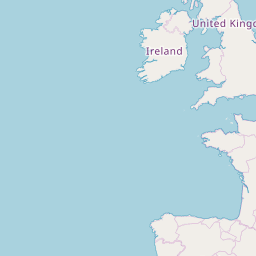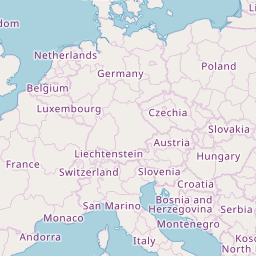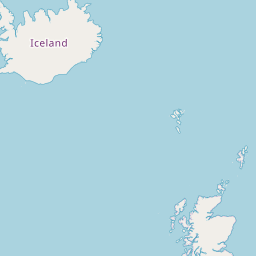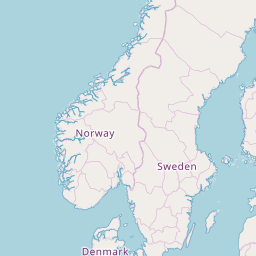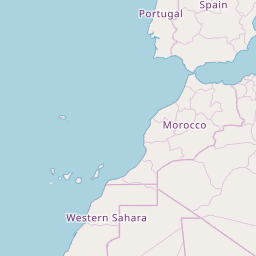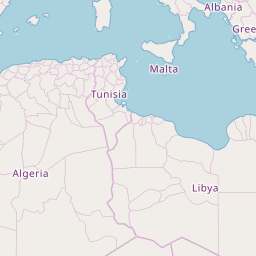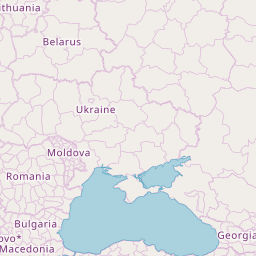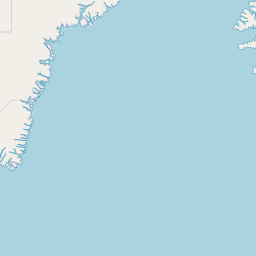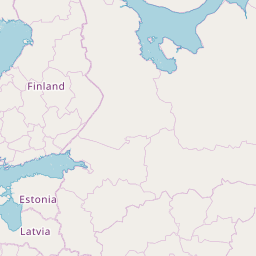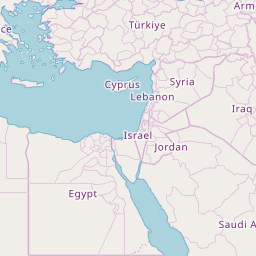### Coordinator

SINTEF - STIFTELSEN FOR INDUSTRIELL OG TEKNISK FORSKNING VED NORGES TEKNISKE HOEGSKOLE
Strindveien 4
7034 Trondheim
Norway

### Participants (3)

THINK3
France
55 Avenue Galline
69100 Villeurbanne

UNIVERSITE DE NICE - SOPHIA ANTIPOLIS
France